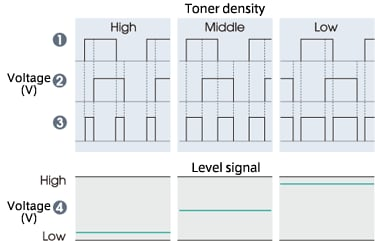﻿

## Toner Density/Quantity Sensors TS Series

### One Component Developer and Two Component Developer

Among the developers used for electrophotographic copying machines, there are one component developers and two component developers. In one component developers, a toner (colored particles) that becomes fixed to the paper is used as the developer. In two component developers, a carrier is mixed in a toner. In addition, there are variations of magnetic and non-magnetic types for both one component and two component developers.

### Roles of the Carrier in Magnetic Two Component Developers

Carriers used in magnetic two component developers have the roles of triboelectrically charging the toner and carrying the toner on the photoreceptor. As the carrier material, iron or ferrite fine particle magnetic powder having a diameter size ranging from 50 to 150μm and being coated with resin for improving environment resistance characteristics and abrasion resistance is commonly used.
A toner has an even smaller particle diameter of around 10μm, and it becomes charged through frictions with the carrier and adsorbed to the photoreceptor, and then transferred to the paper and fixed by a high-heat roller. Generally, a toner is composed of a binder resin, coloring agent, charge control agent, and external additive.
In addition, in a general copying mechanism of two component developers, only the toner is consumed and the carrier is recovered and reused. Therefore, as copying is repeated, the mixture ratio of the toner and carrier gradually becomes carrier rich (the toner density becomes low). Therefore, in order to maintain a good image quality, it is necessary to control an appropriate toner density.

### Toner density Sensing Method in Two Component Magnetic Developers

The toner density sensing and control methods that are currently used can be largely divided into the following two categories:

• 1) a method in which the toner density (image density) is chemically sensed and feedback is applied to each parameter; and
• 2) a method in which the toner density is sensed from the magnetic permeability ratio, volume, or fluidity of the developer and feedback is applied so as to stabilize them.
The former method can be further divided into the following methods:
• 1)-1. a method in which a reference patch image on the photoreceptor is created and the density is sensed by the amount of light reflected from the image; and
• 1)-2. a method in which an image is developed on a clear pseudo photoreceptor using the developer, and the density is sensed by the amount of the light transmitted through the image.
The latter method can also be divided into the following methods:
• 2)-1. a magnetic permeability sensing method using the magnetic permeability ratio of the magnetic body; and
• 2)-2. a method in which the toner amount (volume) and fluidity are sensed
(Figure 1).
Figure 1 Toner density sensing method in two component magnetic developers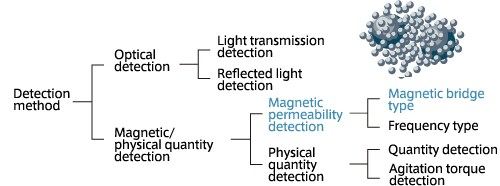### Sensing Method and Driving Principle of the TS Series

Due to the superiority in the shape and cost, the magnetic permeability sensing method of the above 2)-1 is often used for the current mainstream copy machines and laser printers, and the method is also adopted in TDK's TS Series of toner density sensors.

### Areas of Utilization of High Precision Permeability Sensors

The permeability of a developer changes according to the mixture ratio of the carrier (magnetic body such as iron or ferrite) and the toner (non-magnetic body). The excellent sensitivity of the TS Series toner sensors offer benefits that could not be realized by existing sensors in applications other than copy machines and laser printers, including sensing of the existence (contact, proximity) of magnetic bodies or conductors or measurement of the colloid density or dispersion degree. A sample analog output characteristic (Figure 2) and application model (Figure 3) of the TS Series are shown in the following.

Figure 2 Analog output characteristic of the TS Series: Example of permeability detection sensitivity measurement using a ferrite sample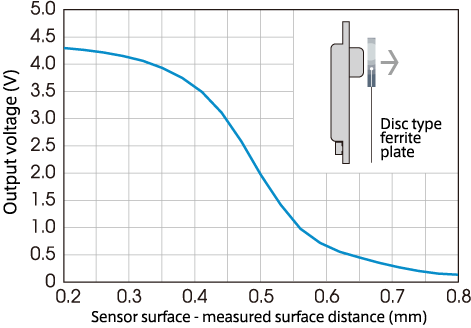Figure 3 TS Series application model
Magnetic body/conductor proximity sensor
(counter/selection switch)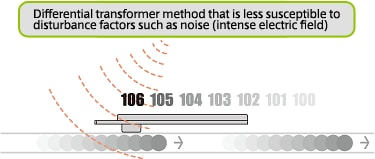Magnetic body/conductor particle density sensor,
dispersion sensor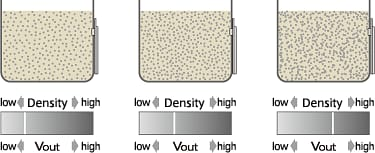### Driving Principle

Figure 4 Principle of a differential transformer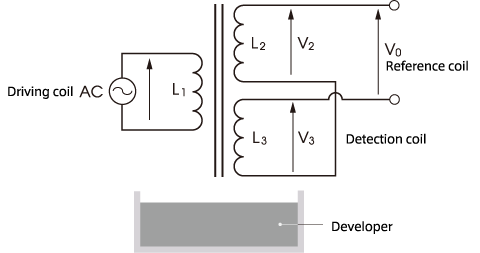The following introduces the technical approaches of TDK, which has established the outstanding output stability of the TS Series.
It is common to use the inductance component of the coil for permeability sensors. This method can be divided into several types, including frequency types and magnetic bridge types. TDK has developed and is offering magnetic bridge type sensors using a differential transformer, and many of them have been adopted by its customers. The operation principle of the sensor is described below.

The coil configuration of a differential transformer is shown in Figure 4. A differential transformer consists of driving coil L1, reference coil L2, and detection coil L3, which are layered concentrically. When L1 is driven at a high frequency,

• differential voltage output V0 = (V2 - V3)

is obtained.

When the output voltages of reference coil L2 and detection coil L3 at the reference developer density are denoted by V20 and V30 respectively and the turns ratio is designed so that V30 becomes equal to V20, the following relationship is established concerning the minute output change △V3 of detection coil L3 in response to the developer density change:

• V0= V20 - (V30+△V3)= - △V3

and the minute change △V3 becomes differential output V0. However, differential output △V3 in response to the toner density near the detection surface is minute in normal developers; therefore, in order to detect it as a voltage variation, extensive amplification processing will become necessary, which will significantly deteriorate the sensor stability.

### Proprietary Phase Discrimination Method

Therefore, TDK has developed a circuit that does not discriminate the voltage but discriminates the phases of △V3 and has applied it to sensors, so as to enhance the amount of output voltage variation without losing stability. In the voltage discrimination method, differential output △V3 is the differential voltage V30 - V20; however, in the phase discrimination method, the phase of differential output △V3 is made to change according to the magnitude of differential voltage V30 - V20.
Namely, the phase P of differential voltage △V3 is made to become

P(V20 - V30) >0 → when the developer density is high
or
P(V20 - V30) (Figure 5).

Figure 5 Phase change of differential output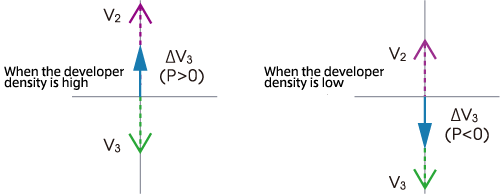For example, if

V20 - V30=0

under the reference concentration, the phase of differential output △V3 reverses at the reference concentration. If reference signal Vr having a phase that is different by 90° is added to differential output △V3 and the phase changes of the combined signal V0 are discriminated by a phase discriminator at this point, it exhibits analogous changes in response to the toner density change, as described in the following (Figure 6).

Figure 6 Analog output by a phase discriminator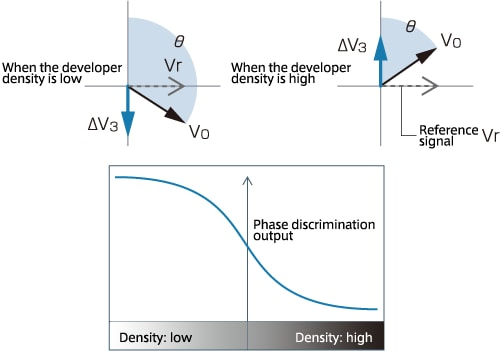### Circuit configuration of the TS Series sensors

The circuit diagram of TS Series using the above method and an output signal example are shown in Figure 7.
In this circuit diagram, all ICs are composed of exclusive OR gates. IC1 operates as a Colpitts oscillator, and drives the coil at approximately 500kHz. IC2 operates as a waveform shaping inverter, and shapes differential outputs into rectangular waves. IC3 operates as a phase comparator.
In addition, C1 is a resonance capacitor, and it minimizes the output offset when there is no developer on the sensor surface. R1 adds the reference signals to the differential signals to maintain the sensor sensitivity at an appropriate level.

Figure 7. The circuit diagram and an output signal example of the TS Series
Driving circuit of the TS Series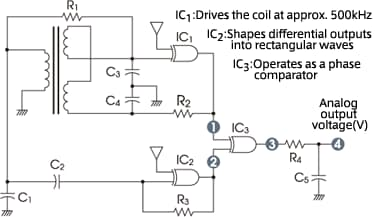Relationship between the toner density and output signal